# Creating Custom Measures• Updated

You can now create your own custom measures within Insights.

To use this feature, bring all the columns you need in the Data area. Then click on the Calculations tab where you can use any of the available operators to build your own measure: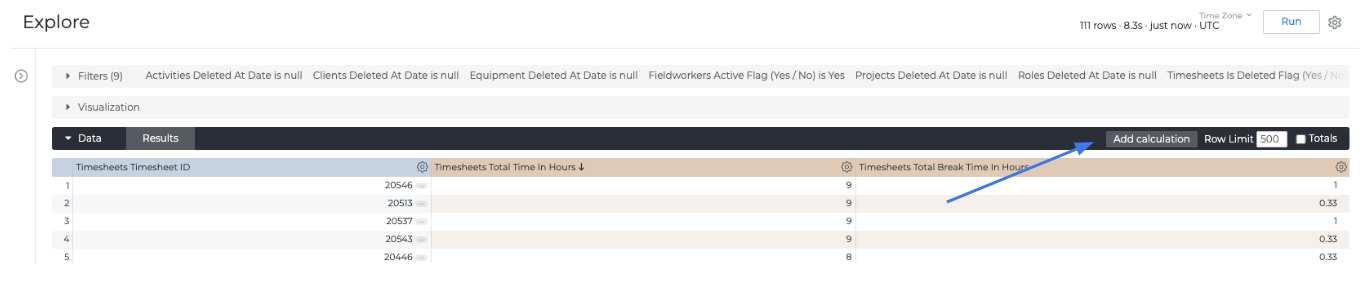Then select a format for the measure (decimal value, currency or percentage):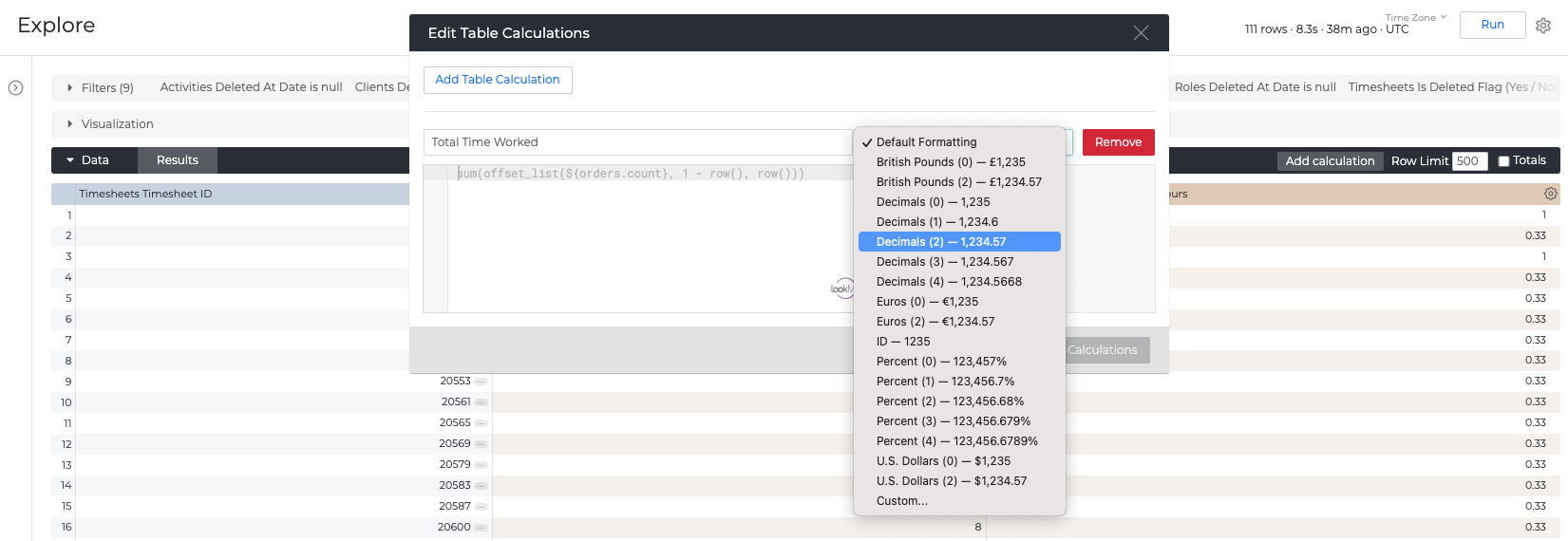Type the columns you want to use in your calculation: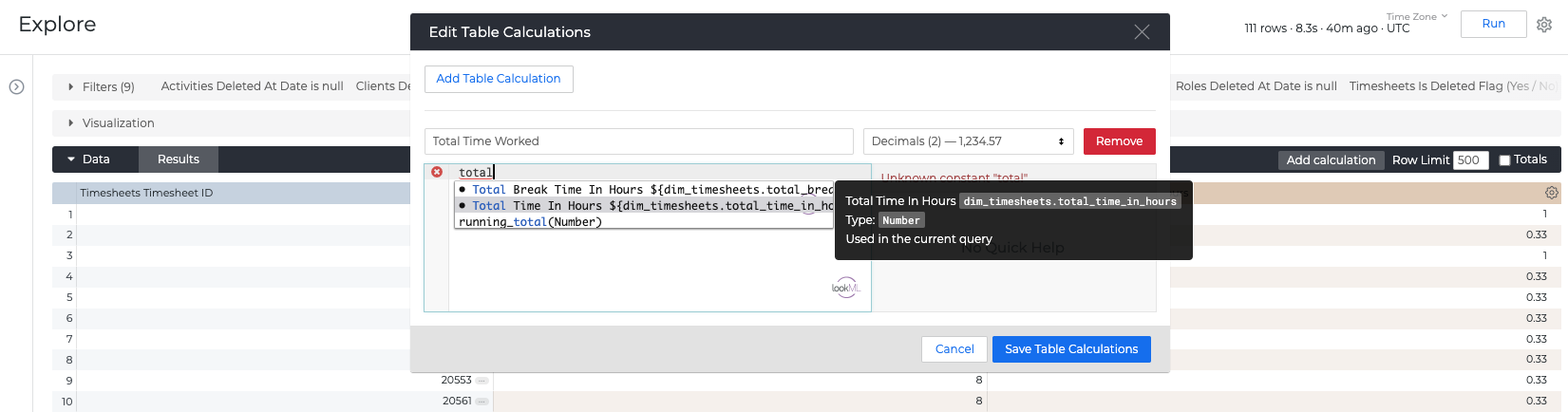Once the formula is defined, click Save Table Calculations.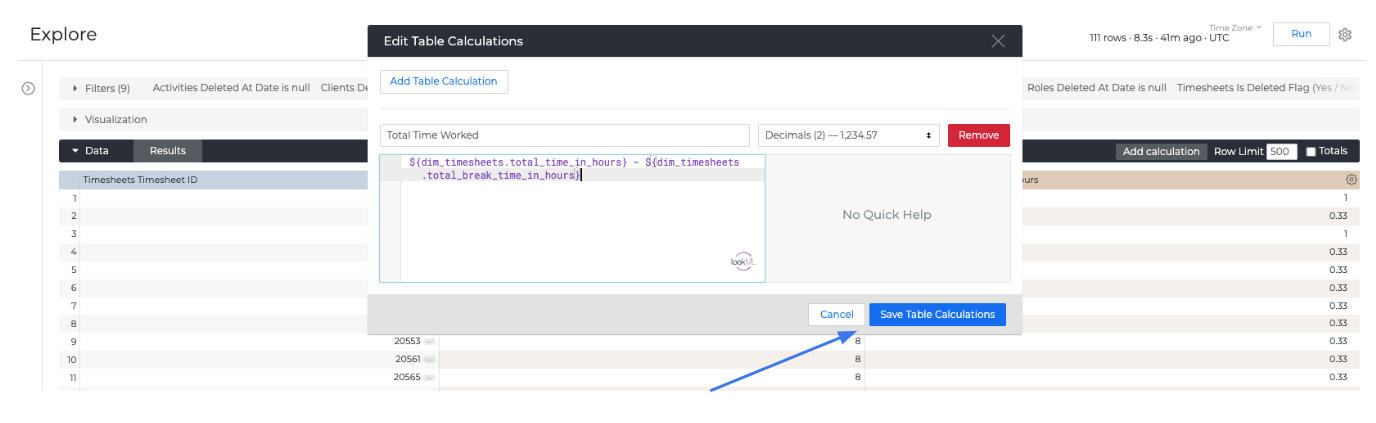Once you save the Table Calculations you will be able to see the green columns in the Report which indicate they are custom measures.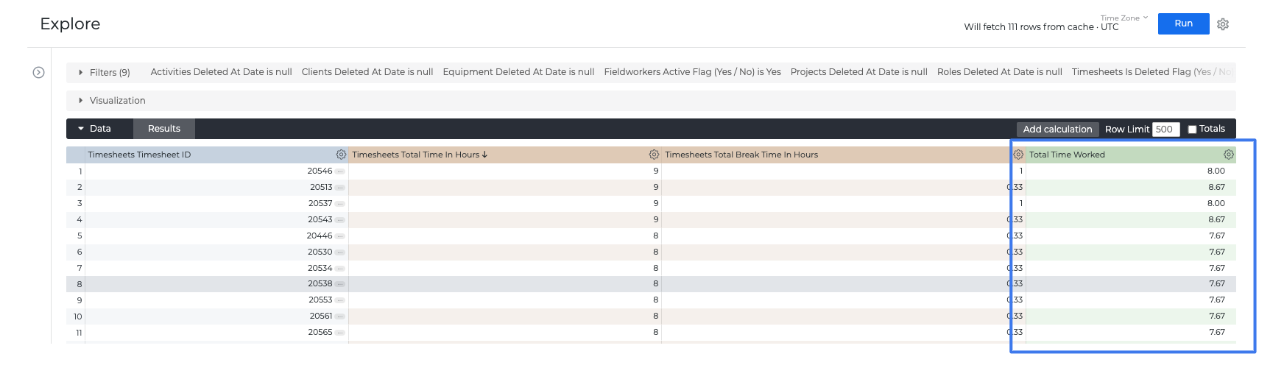If you save this report or dashboard in group reports/dashboards other Insights users will also be able to access this custom measure created by you.

You can add more than 1 Table Calculation in a Report by adding more Table calculations.Operators that can be used are:

 Operator Syntax Purpose + value_1 + value_2 Adds value_1 and value_2. - value_1 - value_2 Subtracts value_2 from value_1. * value_1 * value_2 Multiplies value_1 and value_2. / value_1 / value_2 Divides value_1 by value_2. = value_1 = value_2 Returns Yes if value_1 is equal to value_2, and No otherwise. != value_1 != value_2 Returns Yes if value_1 is not equal to value_2, and No otherwise.

The following comparison operators only can be used with numbers and dates:

 Operator Syntax Purpose > value_1 > value_2 Returns Yes if value_1 is greater than value_2, and No otherwise. < value_1 < value_2 Returns Yes if value_1 is less than value_2, and No otherwise. >= value_1 >= value_2 Returns Yes if value_1 is greater than or equal to value_2, and No otherwise. <= value_1 <= value_2 Returns Yes if value_1 is less than or equal to value_2, and No otherwise.

The following functions only can be used with strings:

 Function Syntax Purpose concat concat(value_1, value_2, ...) Returns value_1, value_2, ..., value_n joined as one string. lower lower(string) Returns string with all characters converted to lowercase. upper upper(string) Returns string with all characters converted to uppercase.

The following functions only can be used with dates:

 Function Syntax Purpose diff_days diff_days(start_date, end_date) Returns the number of days between start_date and end_date. diff_hours diff_hours(start_date, end_date) Returns the number of hours between start_date and end_date. diff_minutes diff_minutes(start_date, end_date) Returns the number of minutes between start_date and end_date. diff_months diff_months(start_date, end_date) Returns the number of months between start_date and end_date. diff_seconds diff_seconds(start_date, end_date) Returns the number of seconds between start_date and end_date. diff_years diff_years(start_date, end_date) Returns the number of years between start_date and end_date. extract_days extract_days(date) Extracts the days from date. extract_hours extract_hours(date) Extracts the hours from date. extract_minutes extract_minutes(date) Extracts the minutes from date. extract_months extract_months(date) Extracts the months from date. extract_seconds extract_seconds(date) Extracts the seconds from date. extract_years extract_years(date) Extracts the years from date. now now() Returns the current date and time.

The following function is a row related offset function. See Dashboard Library -> Orders Reports.

 Function Syntax Purpose offset offset(column, row_offset) Returns the value of row (n + row_offset) in column, where n is the current row number.